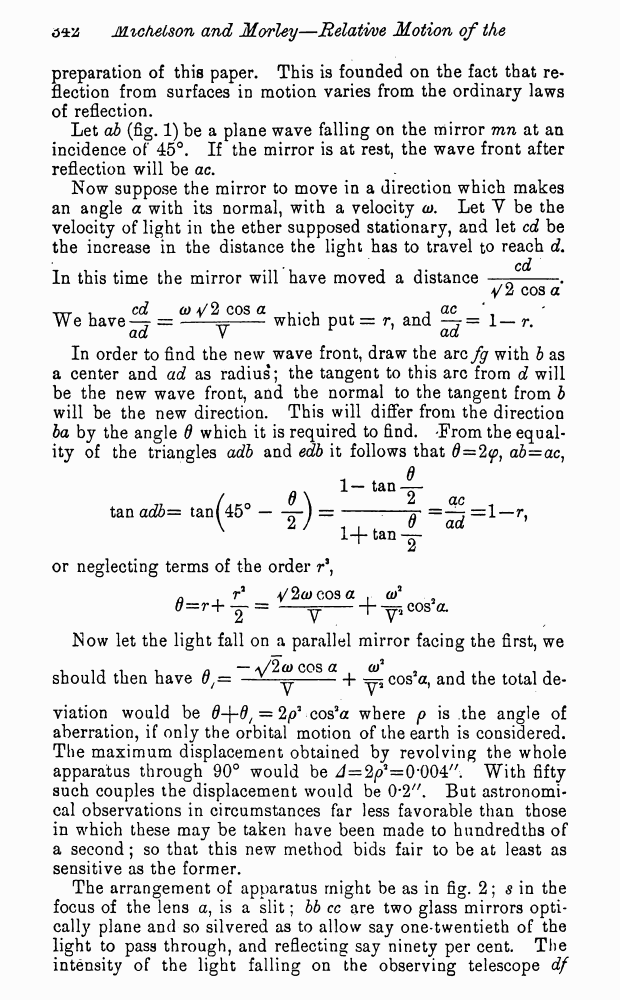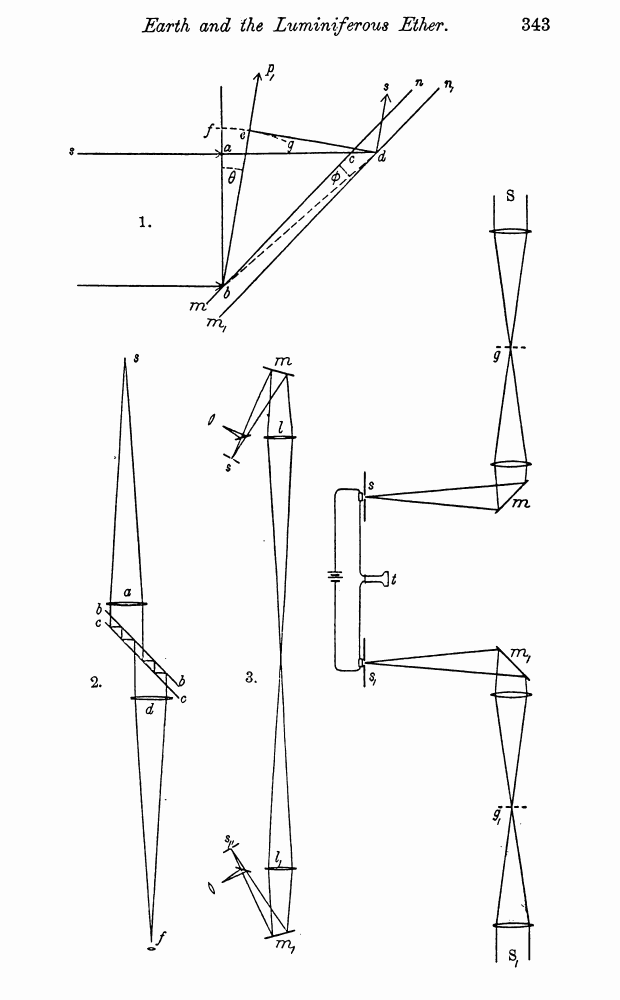# A. Michelson and E. Morley. On the Relative Motion of the Earth and the Luminiferous Ether. // American Journal of Science - Third series - Vol. XXXIV, No. 203. - Nov. 1887.

┬ ĒÓ„ÓļŅ   ─­¾ŃĶÕ ¶Ņ­ņÓ“¹   <<<     č“­ÓĒĶ÷Ó 342   >>>333  334  335  336  337  338  339  340  341  342 343  344  345 preparation of this paper. This is founded on the fact that reflection from surfaces in motion varies from the ordinary laws of reflection. Let ab (fig. 1) be a plane wave falling on the mirror mn at an incidence of 45░. If the mirror is at rest, the wave front after reflection will be ac. Now suppose the mirror to move in a direction which makes an angle a with its normal, with a velocity a). Let Y be the velocity of light in the ether supposed stationary, and let cd be the increase in the distance the light has to travel to reach d. cd In this time the mirror will have moved a distance Ś--. 4/2 cos a ä . cd a) a/2 cos a . . , ^ , ac We haveŚ7 = Ś-which put = r, and Ś7= 1Ś r. ad Y ad In order to find the new wave front, draw the arc fg with b as a center and ad as radius; the tangent to this arc from d will be the new wave front, and the normal to the tangent from b will be the new direction. This will differ from the direction ba by the angle d which it is required to find. 'From the equality of the triangles adb and edb it follows that d=2cosa . of . _ 0 = r+ y = -y--h y5C0Sa* Now let the light fall on a parallel mirror facing the first, we should then have 0f= cos a _j_ ^ COs2a, and the total de viation would be 0+0/ = cos3a where p is the angle of aberration, if only the orbital motion of the earth is considered. The maximum displacement obtained by revolving the whole apparatus through 90░ would be J=2iai=0æ004//. With fifty such couples the displacement would be 0#2". But astronomical observations in circumstances far less favorable than those in which these may be taken have been made to hundredths of a second; so that this new method bids fair to be at least as sensitive as the former. The arrangement of apparatus might be as in fig. 2; s in the focus of the lens a, is a slit; bb cc are two glass mirrors optically plane and so silvered as to allow say one-twentieth of the light to pass through, and reflecting say ninety per cent. The intensity of the light falling on the observing telescope df